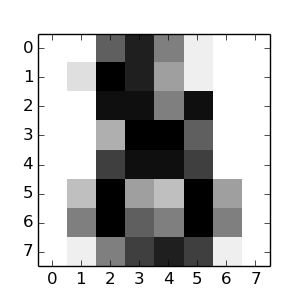This documentation is for scikit-learn version 0.15-gitOther versions

If you use the software, please consider citing scikit-learn.

# The Digit Dataset¶

This dataset is made up of 1797 8x8 images. Each image, like the one shown below, is of a hand-written digit. In order to utilize an 8x8 figure like this, we’d have to first transform it into a feature vector with length 64.

See here for more information about this dataset.Python source code: plot_digits_last_image.py

```print(__doc__)

# Code source: Gaël Varoquaux
# Modified for documentation by Jaques Grobler
# License: BSD 3 clause

from sklearn import datasets

import matplotlib.pyplot as plt

#Load the digits dataset
digits = datasets.load_digits()

#Display the first digit
plt.figure(1, figsize=(3, 3))
plt.imshow(digits.images[-1], cmap=plt.cm.gray_r, interpolation='nearest')
plt.show()
```

Total running time of the example: 0.13 seconds ( 0 minutes 0.13 seconds)